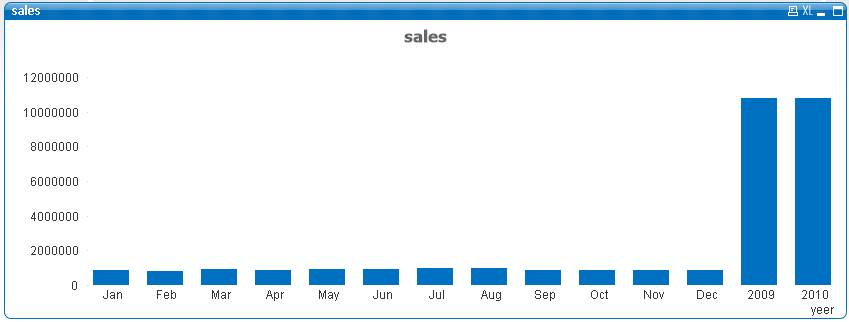# QlikView App Development

Discussion Board for collaboration related to QlikView App Development.

Highlighted
New Contributor II

## Sorting a calculated dimension in a bar chart

Hello Guys,

I am having issue while sorting a calculated dimension in a bar chart. I am using calculated dimension which produce yearly data point as well as monthly data points(only for the last year).I need to sort the data field firstly by year and then by month. I have tried using match function in expression in sort tab but it's not working for me.

The following calculated dimension I am using :

=if(Year <> vMaxYear,Year,Month)

So, in case of last year it will show data monthly wise otherwise yearly.

Please refer to below graph for output. So, I need to have data like this 2009, 2010, jan , feb, mar, apr...... dec.Any help would be appreciated.

Thanks & Regards

Harsh

Tags (2)
1 Solution

Accepted SolutionsMVP

## Re: Sorting a calculated dimension in a bar chart

May be try a sort expression like this with ascending sort:

RangeSum(Year, Num(Month)*10000)

6 RepliesMVP

## Re: Sorting a calculated dimension in a bar chart

May be try a sort expression like this with ascending sort:

RangeSum(Year, Num(Month)*10000)MVP

## Re: Sorting a calculated dimension in a bar chart

maybe sorting (Sort tab) only by this expression

=if(Year <> vMaxYear,Year,Month*10000)

New Contributor II

## Re: Sorting a calculated dimension in a bar chart

Thanks Sunny for the quick reply. It worked like charm.I just have a little doubt how we are using rangesum for sorting purpose here? Normally, we use it for rolled up sum, avg etc... if you could explain it a bit that would be much help for better understanding.

New Contributor II

## Re: Sorting a calculated dimension in a bar chart

Thanks Massimo for immediate respone. your solution did work too but by multiplying the month with 10000 it is changing the data points values into 10000, 20000, 300000...... .MVP

## Re: Sorting a calculated dimension in a bar chart

The only reason I used RangeSum is because there might be nulls which I thought might create problems. I could have just given you this:

Year + Num(Month) * 10000

But if either Year or Num(Month) * 10000 are null, the whole row will turn into null. Whereas,

RangeSum(Year, Num(Month)*10000)

will take care of nulls.

So, RangeSum is not just used for rolled up sum (not sure what that means), but it is also used to Sum two expressions where one can be null at times. Other longer alternatives can be like this

Alt(Year, 0) + Alt(Num(Month) * 10000, 0)

or

If(Len(Trim(Year)) = 0, 0, Year) + If(Len(Trim(Num(Month))) = 0, 0, Num(Month) * 10000)

All of these are safety nets and not necessarily needed. But why not use a simple RangeSum instead of + if it is more robust, right?MVP

## Re: Sorting a calculated dimension in a bar chart

You can also read about some of what I explained here under the header 'Nulls in Basic Maths'

QlikView Addict: Handling Nulls in QlikView